Midterm 1 Preparation

# Midterm 1: Version A

1. Evaluate: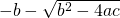if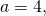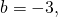and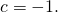2. Solve for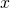in the equation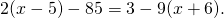3. Isolate the variable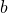in the equation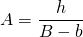.
4. Solve forin the equation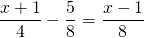.
5. Write an equation of the vertical line that passes through the point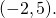6. Find the equation that has a slope of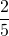and passes through the point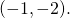7. Find the equation of the line passing through the points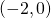and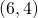.
8. Graph the relation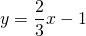.

For questions 9 to 11, find each solution set and graph it.

1.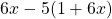>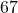2.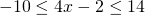3.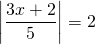4. Graph the relation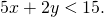5. Find two numbers such that 5 times the larger number plus 3 times the smaller is 47, and 4 times the larger minus twice the smaller is 20.
6. Karl is going to cut a 36 cm cable into 2 pieces. If the first piece is to be 5 times as long as the second piece, find the length of each piece.
7.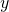varies jointly with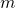and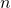and inversely with the square of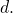If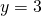when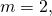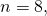and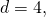find the constant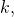then use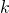to findwhen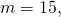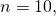and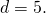<a class=”internal” href=”/intermediatealgebraberg/back-matter/midterm-one-version-a-answer-key/”>Midterm 1: Version A Answer Key